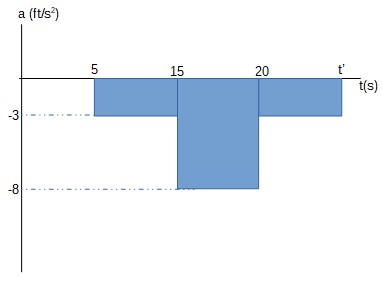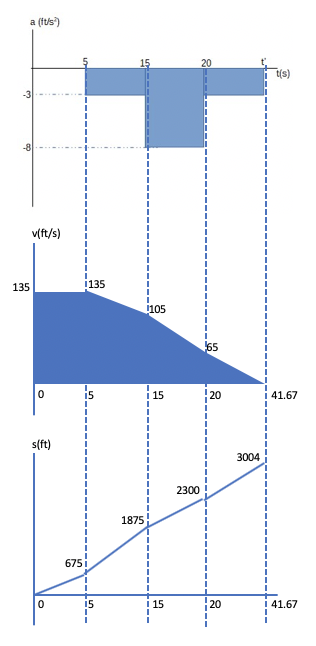# An airplane lands on the straight runway, originally traveling at 135 ft/s when s = 0. If it is...

## Question:

An airplane lands on the straight runway, originally traveling at 135 ft/s when s = 0. If it is subjected to the deceleration shown, determine the time t' needed to stop the plane and construct the v-t and s-t graphs for the motion.## Velocity:

Velocity is defined as the speed of an object and its direction. On the other hand, the change in velocity is mathematically defined as the integral of the product of the acceleration and time as shown below.

{eq}\Delta v = \int a \,dt {/eq}

The integral function can be simplified by a summation function.

Given that the airplane has an initial velocity of 135 ft/s, the total change in velocity must be

{eq}\Delta v = 0 - 135 = -135 \, \dfrac {ft}{s}...(1)\\ {/eq}

The sum of the product of acceleration and time is:

{eq}\int a \, dt = \sum (a)(\Delta t)\\ \int a \, dt = (0)(5-0) + (-3)(15-5) + (-8)(20-15) + (-3)(t' - 20)\\ \int a \, dt = (-3)(10) + (-8)(5) + (-3)(t' - 20)\\ \int a \, dt = -10 -3t'...(2)\\ {/eq}

Solving equations (1) and (2):

{eq}-135 = -10 -3t'\\ \boxed {t' = 41.67\, sec}\\ {/eq}

At t = 5:

{eq}v_5 = v_o + a_5t\\ v_5 = 135 + (0)(5-0)\\ \boldsymbol {v_5 = 135 \, ft/s}\\ {/eq}

{eq}x_5 = x_o + 0.5(v_o +v_5)t\\ x_5 = 0 + 0.5(135 + 135)(5)\\ \boldsymbol {x_5 = 675 \, ft}\\ {/eq}

At t = 15:

{eq}v_{15} = v_{5} + a_{15}t\\ v_{15} = 135 + (-3)(15-5)\\ \boldsymbol {v_{15} = 105 \, ft/s}\\ {/eq}

{eq}x_{15} = x_{5} + 0.5(v_{5} +v_{15})t\\ x_{15} = 675 + 0.5(135 + 105)(15-5)\\ \boldsymbol {x_{15} = 1875 \, ft}\\ {/eq}

At t = 20:

{eq}v_{20} = v_{15} + a_{20}t\\ v_{20} = 105 + (-8)(20-15)\\ \boldsymbol {v_{20} = 65 \, ft/s}\\ {/eq}

{eq}x_{20} = x_{15} + 0.5(v_{15} +v_{20})t\\ x_{20} = 1875 + 0.5(105 + 65)(20-15)\\ \boldsymbol {x_{20} = 2300 \, ft}\\ {/eq}

At t = 41.67:

{eq}v_{41.67} = v_{20} + a_{41.67}t\\ v_{41.67} = 65 + (-3)(41.67-20)\\ \boldsymbol {v_{41.67} = 0 \, ft/s}\\ {/eq}

{eq}x_{41.67} = x_{20} + 0.5(v_{20} +v_{41.67})t\\ x_{41.67} = 2300 + 0.5(65 + 0)(41.67-20)\\ \boldsymbol {x_{41.67} = 3004 \, ft}\\ {/eq}